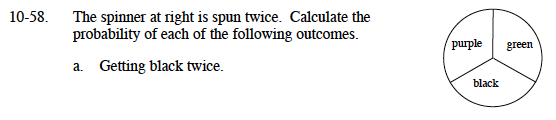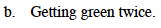### Home > MC1 > Chapter 10 > Lesson 10.1.5 > Problem10-58

10-58.What is the probability of getting black once? Multiply that by itself to find the probability of getting black twice.

$\left(\frac{1}{3}\right)\left(\frac{1}{3}\right)=\frac{1}{9}$Is it any more or less likely that someone will get green over black?

Use the eTool below to test the probability.
Click the link at right for the full version of the eTool: MC1 10-58 HW eTool